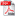# MODELING OF CONSTANT VOLUME COMBUSTION OF PROPELLANTS FOR ARTILLERY WEAPONS

Autor/autori: Lt.col. lect. dr.ing. Daniel ANTONIE, Col.(r). prof.as. dr.ing. Sorin GHEORGHIAN, Col.(r). C.S.II dr.ing. Nicolae MARUNTELU

Abstract: Combustion of artillery propellants is a very complex physical and chemical process which depends on a large number of parameters. This paper presents a mathematical model consisting of the Nobel-Abel equation of state, the energy conservation equation, the equation of conservation of mass and burning rate law. The system of equations associated with this mathematical model is solved numerically using a computer program. Finally it presents theoretical results obtained with this program for M30 triple-base seven perforated granular propellant, compared with experimentally results measured using closed vessel technique

Keywords: combustion, solid propellant, closed vessel, mathematical model, computer programDOWNLOAD PDF